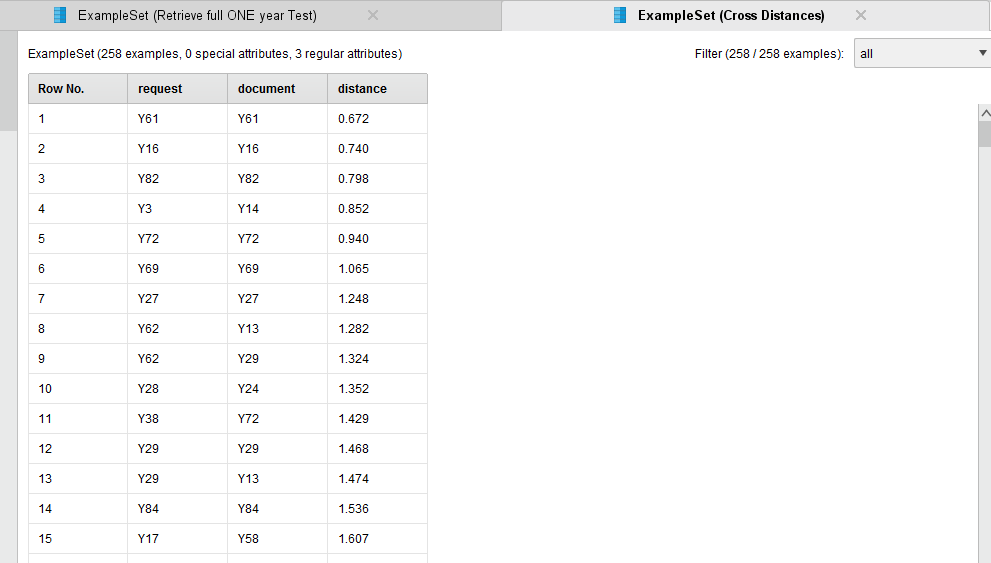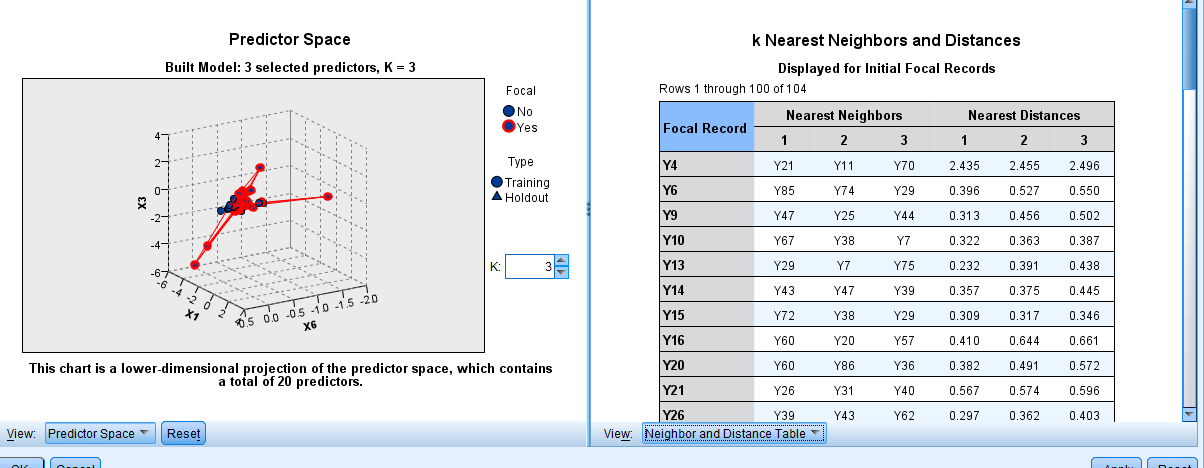# how to get the ranks of unlabeled case using K-NN

Member Posts: 44Contributor I
edited November 2018 in Help
Hi all, I have unlabeled data and want to get rank of its nearest cases so I can compare it with them, Its credit rating problems so I have unlabeled customers and want to know the nearest neighbor of them by ranking or how close they are to the good or bad customers

• RapidMiner Certified Analyst, RapidMiner Certified Expert, Member Posts: 578Unicorn
It sounds like you aren't needing the k-NN operator, but rather the Cross Distances.  (Other similarity operators are also useable).
Have a look at this sample process using the Golf dataset.
`<?xml version="1.0" encoding="UTF-8" standalone="no"?><process version="7.0.000">  <context>    <input/>    <output/>    <macros/>  </context>  <operator activated="true" class="process" compatibility="6.0.002" expanded="true" name="Process">    <process expanded="true">      <operator activated="true" class="retrieve" compatibility="7.0.000" expanded="true" height="68" name="Golf" width="90" x="45" y="187">        <parameter key="repository_entry" value="//Samples/data/Golf"/>      </operator>      <operator activated="true" class="generate_id" compatibility="7.0.000" expanded="true" height="82" name="Generate ID" width="90" x="179" y="238">        <parameter key="create_nominal_ids" value="true"/>        <description align="center" color="transparent" colored="false" width="126">Using nominal IDs just to demo.</description>      </operator>      <operator activated="true" class="retrieve" compatibility="7.0.000" expanded="true" height="68" name="Retrieve Golf-Testset" width="90" x="45" y="34">        <parameter key="repository_entry" value="//Samples/data/Golf-Testset"/>      </operator>      <operator activated="true" class="subprocess" compatibility="7.0.000" expanded="true" height="82" name="Get only 1 record." width="90" x="179" y="34">        <process expanded="true">          <operator activated="true" class="sample" compatibility="7.0.000" expanded="true" height="82" name="Sample" width="90" x="45" y="34">            <parameter key="sample_size" value="1"/>            <list key="sample_size_per_class"/>            <list key="sample_ratio_per_class"/>            <list key="sample_probability_per_class"/>          </operator>          <operator activated="true" class="select_attributes" compatibility="7.0.000" expanded="true" height="82" name="Select Attributes" width="90" x="179" y="34">            <parameter key="attribute_filter_type" value="single"/>            <parameter key="attribute" value="Play"/>            <parameter key="invert_selection" value="true"/>            <parameter key="include_special_attributes" value="true"/>          </operator>          <operator activated="true" class="generate_id" compatibility="7.0.000" expanded="true" height="82" name="Generate ID (2)" width="90" x="313" y="34"/>          <connect from_port="in 1" to_op="Sample" to_port="example set input"/>          <connect from_op="Sample" from_port="example set output" to_op="Select Attributes" to_port="example set input"/>          <connect from_op="Select Attributes" from_port="example set output" to_op="Generate ID (2)" to_port="example set input"/>          <connect from_op="Generate ID (2)" from_port="example set output" to_port="out 1"/>          <portSpacing port="source_in 1" spacing="0"/>          <portSpacing port="source_in 2" spacing="0"/>          <portSpacing port="sink_out 1" spacing="0"/>          <portSpacing port="sink_out 2" spacing="0"/>        </process>      </operator>      <operator activated="true" class="cross_distances" compatibility="7.0.000" expanded="true" height="103" name="Cross Distances" width="90" x="313" y="85">        <parameter key="only_top_k" value="true"/>        <parameter key="k" value="3"/>      </operator>      <operator activated="true" class="select_attributes" compatibility="7.0.000" expanded="true" height="82" name="Select only label" width="90" x="447" y="238">        <parameter key="attribute_filter_type" value="subset"/>        <parameter key="attributes" value="id|Play"/>        <parameter key="include_special_attributes" value="true"/>      </operator>      <operator activated="true" class="join" compatibility="7.0.000" expanded="true" height="82" name="Join to Request" width="90" x="447" y="34">        <parameter key="use_id_attribute_as_key" value="false"/>        <list key="key_attributes">          <parameter key="request" value="id"/>        </list>        <description align="center" color="transparent" colored="false" width="126">This join is just to get the original data back rather than just the ID.</description>      </operator>      <operator activated="true" class="join" compatibility="7.0.000" expanded="true" height="82" name="Join to Reference" width="90" x="581" y="187">        <parameter key="use_id_attribute_as_key" value="false"/>        <list key="key_attributes">          <parameter key="document" value="id"/>        </list>        <description align="center" color="transparent" colored="false" width="126">When the result is joined with the original Reference dataset then the label is used.</description>      </operator>      <connect from_op="Golf" from_port="output" to_op="Generate ID" to_port="example set input"/>      <connect from_op="Generate ID" from_port="example set output" to_op="Cross Distances" to_port="reference set"/>      <connect from_op="Retrieve Golf-Testset" from_port="output" to_op="Get only 1 record." to_port="in 1"/>      <connect from_op="Get only 1 record." from_port="out 1" to_op="Cross Distances" to_port="request set"/>      <connect from_op="Cross Distances" from_port="result set" to_op="Join to Request" to_port="left"/>      <connect from_op="Cross Distances" from_port="request set" to_op="Join to Request" to_port="right"/>      <connect from_op="Cross Distances" from_port="reference set" to_op="Select only label" to_port="example set input"/>      <connect from_op="Select only label" from_port="example set output" to_op="Join to Reference" to_port="right"/>      <connect from_op="Join to Request" from_port="join" to_op="Join to Reference" to_port="left"/>      <connect from_op="Join to Reference" from_port="join" to_port="result 1"/>      <portSpacing port="source_input 1" spacing="0"/>      <portSpacing port="sink_result 1" spacing="0"/>      <portSpacing port="sink_result 2" spacing="0"/>    </process>  </operator></process>`
• Member Posts: 44Contributor I
thanks a lot for quick reply and help, I will try to get more understanding of it and will give you feedback, but want to know is that based on the K-NN? as i can see the number of neighours and distance so on

also if you have more tutorial on that process i will be pleased to tell me, I will give you feedback soon thanks again apperciate it
• Member Posts: 44Contributor I
it just give me the distance , I donot distance from what? also I have 516 case so I found huge distance

I want to enter the unlabeled cases to be assigned for the most close similar case, using nearest neighbour, I donot know how to do it
it is something like that

https://dato.com/learn/userguide/nearest_neighbors/nearest_neighbors.html

thanks a gain
• RapidMiner Certified Analyst, RapidMiner Certified Expert, Member Posts: 578Unicorn
If you are wanting to assign it to the value of the nearest value then k-NN with k = 1 is what you are looking for.  If it is to look at the nearest 3 cases then k-NN with k = 3 is what you would like, this would assign the missing labels to the closest record where you do have a value by weight vote.
As you are assigning it to the missing labels, maybe try the operator 'Impute Missing Values' with k-NN inside it.

If what you are looking is what the k closest records to your sample record is then the similarity operators (such as Cross Distance) are what you need.

What do you want to happen in your process?• Member Posts: 44Contributor I
am really thankfull for your feedback and keeping up with me, actually my last step in my research depends on that step so hope to help me,

first of all, I want to enter training data to make the model train on ( Neural network or K-nn) whatever is okie,

then enter the unlableled data ( same as exampleset but with missing values in the label column)

the result to give me the best 5 closest and similar cases from the labeled data ( Exampleset) , so I donot know the right operator to use, secondly the results appear like that using the cross distancebut i want it to appear in something like that ( i used spss modeler but there isno predication in it )